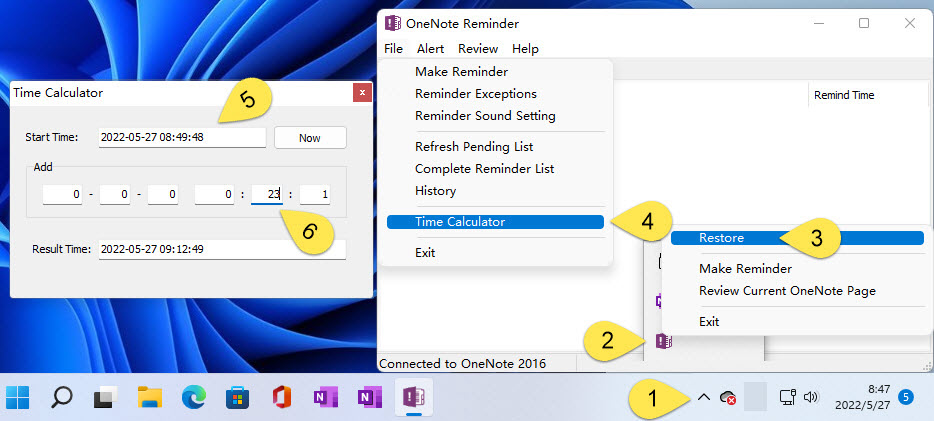### OneNote Time Calculator – Calculates the Moment after a Specified Interval

When using the OneNote reminder tool, it is sometimes necessary to remind you of a series of consecutive events.

For example, after a certain moment of reminder to do something, and then dozens of minutes after the follow-up, another reminder to do continuous related things.

## Calculates the Next Consecutive Reminder Moment

Although, many times, we can do oral calculations to calculate the moment of the next event. But because time is not calculated in decimal, but in 60. So, a lot of times the calculation becomes less so easy.
The OneNote Reminder Tool, now available as a time calculator, is available for this purpose to calculate the moment of the next event.

### OneNote Time Calculator